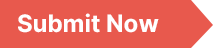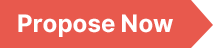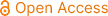### Mathematics and Computer Science

Archive
• 2023, Volume 8

• 2022, Volume 7

• 2021, Volume 6

• 2020, Volume 5

• 2019, Volume 4

• 2018, Volume 3

• 2017, Volume 2

• 2016, Volume 1

Submit a Manuscript

Publishing with us to make your research visible to the widest possible audience.Propose a Special Issue

Building a community of authors and readers to discuss the latest research and develop new ideas.### Analysis and Optimization of Improved Index Calculus Algorithm

IC (Index Calculus) algorithm is the most effective probability algorithm for solving discrete logarithm of finite prime fields, and IICA (improved Index Calculus algorithm) is an improved algorithm based on IC in the third stage. The essence of IICA is to convert the number required to solve the discrete logarithm into the product of the power of prime factors, and then multiply every prime factor larger than the smooth bound by a smooth number approximating a large prime p from the right end, that is, to perform congruence transformation for every prime factor larger than the smooth bound. If all prime factors larger than the smooth bound fall within the smooth bound, the number required to solve the discrete logarithm is successfully solved. Unfortunately, given a large prime number p, some prime factors do not have congruent transformations of smooth numbers. For this, this paper analyzes the features of the IICA algorithm, based on the characteristics of IICA algorithm, is given when the IICA algorithm cannot undertake congruence transformation termination conditions, namely when ⌈ p/pi ⌉ not smooth algorithm is terminated, where pi is greater than a smooth boundary element factor. According to the ⌈ p/pi ⌉ not smooth algorithm was terminated when judging conditions, optimized the IICA algorithm, and the correctness of the optimization algorithm is verified by an example.

Discrete Logarithm, Decomposition Base, Smooth Boundary, Factorization, Prime Finite Fields

Hu Jianjun. (2023). Analysis and Optimization of Improved Index Calculus Algorithm. Mathematics and Computer Science, 8(2), 57-61. https://doi.org/10.11648/j.mcs.20230802.14